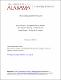## Fibonacci sequences in groupoids2012
Springer
##### Abstract

In this article, we consider several properties of Fibonacci sequences in arbitrary groupoids (i.e., binary systems). Such sequences can be defined in a left-hand way and a right-hand way. Thus, it becomes a question of interest to decide when these two ways are equivalent, i.e., when they produce the same sequence for the same inputs. The problem has a simple solution when the groupoid is flexible. The Fibonacci sequences for several groupoids and for the class of groups as special cases are also discussed. 2000 Mathematics Subject Classification: 20N02; 11B39.

##### Keywords
fibonacci sequences, groupoid, flexible, wrapped around, Mathematics, Applied, Mathematics
##### Citation
Han, J., Kim, H., Neggers, J. (2012): Fibonacci Sequences in Groupoids. Advances in Difference Equations. Volume 19.